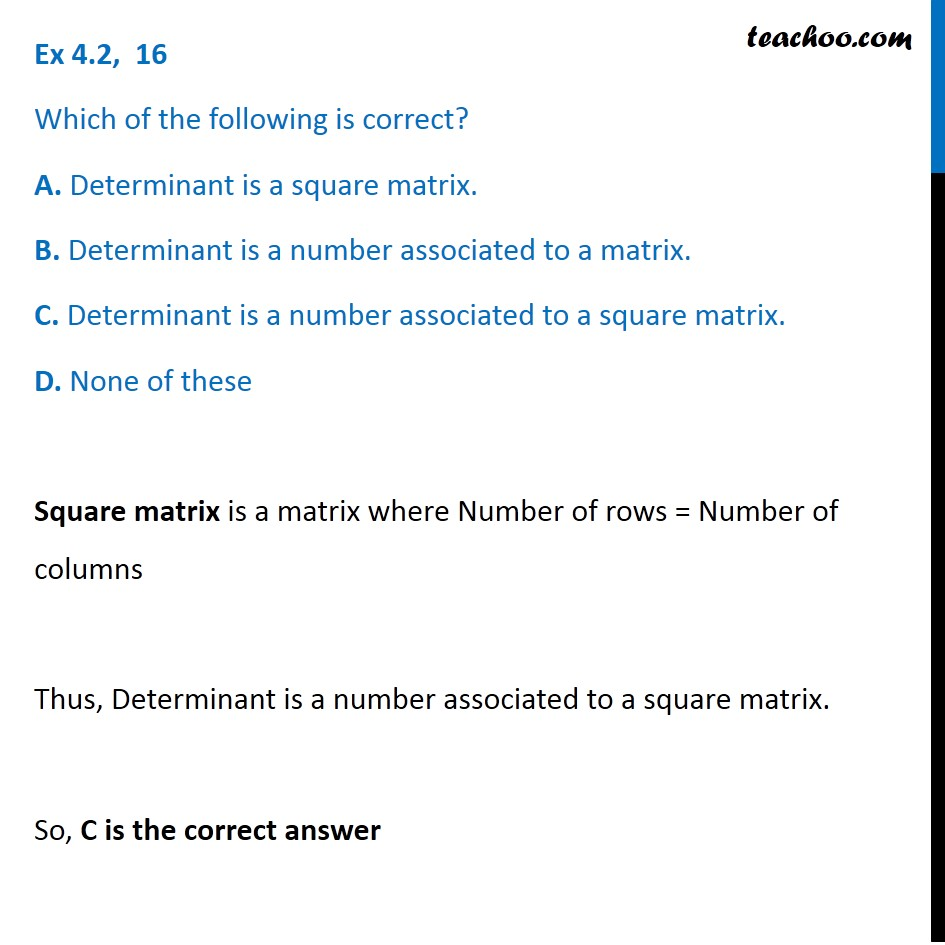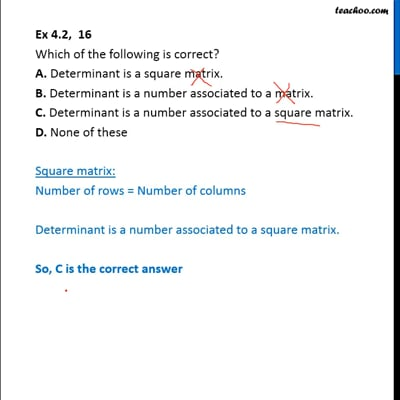Properties of Determinant

Chapter 4 Class 12 Determinants
Serial order wiseThis video is only available for Teachoo black users

Learn in your speed, with individual attention - Teachoo Maths 1-on-1 Class

### Transcript

Question 16 Which of the following is correct? A. Determinant is a square matrix. B. Determinant is a number associated to a matrix. C. Determinant is a number associated to a square matrix. D. None of these Square matrix is a matrix where Number of rows = Number of columns Thus, Determinant is a number associated to a square matrix. So, C is the correct answer